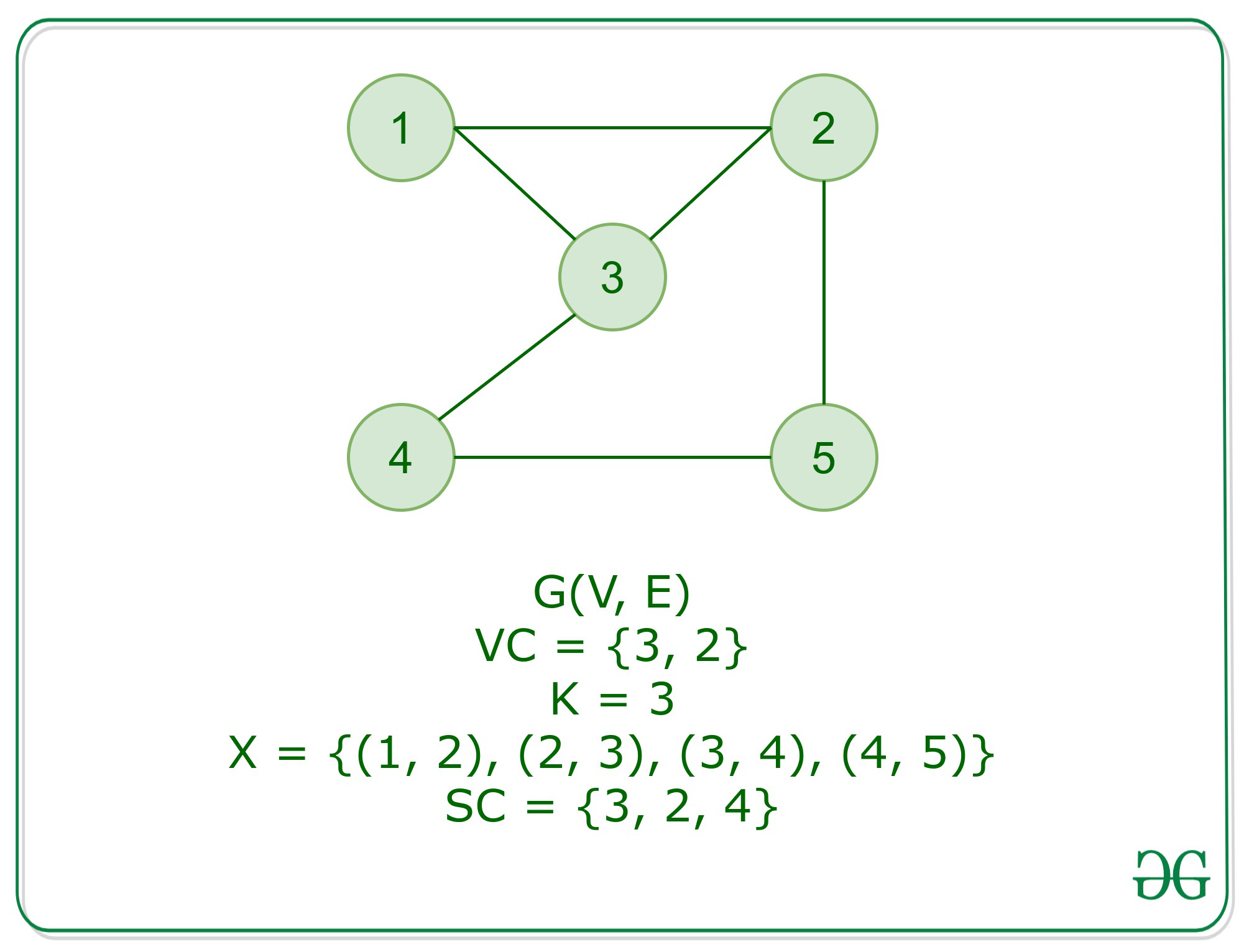GFG App
Open AppBrowser
Continue

# Set cover is NP Complete

Problem: Given a ground Set X, an integer k, and a collection of subsets Si of X, the problem is to identify if there exists a collection of subsets whose union is X, with size at most k.

Proof: An instance of the problem is an input specified to the problem. An instance of the Set Cover problem is a Ground set X, an integer k and a collection of subsets Si formed out of X. Since an NP-complete problem, by definition, is a problem which is both NP and NP-Hard, the proof or statement that a problem is NP-Complete consists of two parts:

1. The problem itself is NP-Complete.
2. All other problems in NP class can be polynomial-time reducible to that. (B is poly-time reducible to C).

If the only second condition is satisfied, the problem is called NP-Hard. But it is not possible to reduce every NP problem into another NP problem to show its NP-Completeness all the time. That is why if we want to show a problem is NP-complete, prove that the problem is in NP and any NP-Complete problem is reducible to that. Thus, it can be verified that the set cover problem is NP-Complete using the following propositions:

1. Set Cover is in NP: If any problem is in NP, then given a ‘certificate’, which is a solution to the problem and an instance of the problem ( a collection of subsets, C of size k), we will be able to identify (whether solution is correct or not) certificate in polynomial time. This can be done by:
Provide a collection C of subsets of size k, we can iterate over each element in the subsets of collection and mark the elements in X which are covered. At the end, no elements should be uncovered in X.
This takes polynomial time with respect to the number of subsets in X. Hence, Set Cover is in NP.
2. Set Cover is NP-Hard: In order to prove set cover is NP Hard, we will perform a reduction from a known NP-Hard problem, that is, vertex cover to set cover problem.For the vertex cover problem, we have as input a graph G = (V, E) and an integer k. Now, let the ground set
• X = E, that is the set of edges in G.
• Subset Su for every vertex u in V, contains the edges incident to u.Now, the following two propositions hold:

• Let us consider that k sets Su1, Su2……Suk cover the ground set X, then every edge e in E is adjacent to minimum one vertex from u1…uk, Therefore forming a vertex cover of size k.
• Let us consider vertices u1…uk form a vertex cover, then Su1 covers all edges incident at u1. Hence, the collection of sets Su1, Su2…Suk form set cover covering X.

Conclusion:

The set-cover is NP and NP-Hard. Therefore, the set-cover is NP-Complete.

My Personal Notes arrow_drop_up